# The Decimal Square

### A fresh look at Multiplication Tables

Allan Lawson describes how he devised a system of dynamic vector patterns for use in teaching the multiplication tables.

The apparent universal ability for young children to manipulate small groups of dot patterns in their mindʼs eye, in solving addition and subtraction problems led me directly to a new challenge; how to find a method for solving the basic multiplication facts using dot patterns.

I realized this challenge might require a completely different approach compared to that of the Math Square which I developed earlier for teaching the addition facts. As I discovered, the solution to the challenge did in fact involve the Math Square – but not before some mathematical developments had been applied first.

Two stages were required – the first of these developments involved the transformation of the Math Square into a more precise numeral system. The second stage involved transforming this new numeral system into an arithmetic system to display the multiplication tables.

### The First Stage – a new numeral system

Math Square numerals are formed by groups of dots in various patterns. These patterns are systematically built in a 3 by 3 square format, representing the numbers 1 to 10. Typical dot patterns for 1 to 10 would look like this:-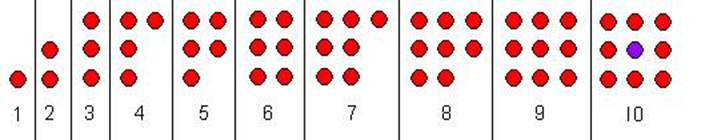[the purple dot at the centre of the ten dot pattern represents two dots, or counters, placed one above the other]

The first stage in achieving my goal of solving the multiplications using patterns was to transform these groups of dots into an ordinal numeral system.
Instead of recognizing each individual pattern as a whole group of dots (what I term “group collection”) – for example the figure below is recognized as 8: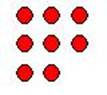– If we label each counter in the Math Square with a designated fixed number from 0 to 9, we only need to know the position of any single counter to know its designated number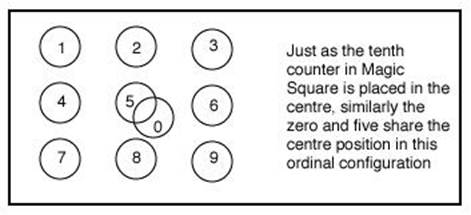For example, we now know that the bottom left-hand counter is 7, even if none of the counters are actually labeled with numbers. We know it is 7 because of its position within the framework of the square format.Now if we reduce the circular counters to numbered points on a framework of 3 horizontal and 3 vertical lines, we would have a number framework – which I call the Decimal Square:-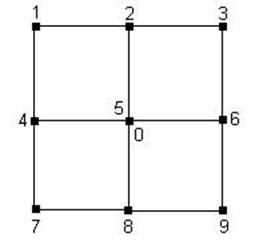This configuration was an important step in my overall goal.

The Arabic numerals labeling each intersection of the Decimal Square become redundant, as each intersection has its fixed numerical value from 0 to 9 (called “number positions”). They are shown in the diagram above for indication purposes only.

So the decimal square is a positional numeral system. To demonstrate this, a series of squares could be arranged horizontally, each square in ascending powers of 10, to represent any number.

For example 53, 370 would be represented as: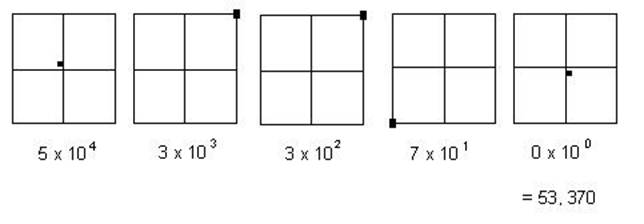The Decimal Square numeral system can now be adapted and form a solution to our goal of creating a system of algorithms to solve multiplications using patterns – this being the second stage of development referred to earlier.

### The Second Stage – Vector-based Multiplication Tables

Finding patterns in multiplication tables is not new; what we are aiming to do, however, is to discover a new kind of pattern – one formed by movement from one number position to another in a positional numeral system. This is where the Decimal Square comes to the rescue.

If we re-introduce Arabic numerals into the Decimal Square and label each number position with its number, we could then explore the patterns of lines* formed by

connecting each number position in turn as we proceed through the multiplication tables. In the process, we will be developing the Decimal Square into an arithmetic system for solving our multiplication pattern question.

[* the connecting lines being referred to as “vectors” – a line that indicates connectivity and direction]

The Decimal Square, labeled with its fixed unit number positions, looks like this:

The Decimal SquareNow, taking as an example the 3 times table. Starting from the 3 position on the square (1×3), vectors are drawn in green on the square, joining each unit number position as we move through the table from 1x 3 to 11 x 3:We discover a simple pattern of parallel green lines formed by the vectors; 3 pointing south, and one diagonal pointing North East, where the pattern repeats itself from the 11×3 position. And we also see a simple numerical progression where we write the tens values (in red) in their relevant unit positions.

Let us examine the vectors formed in another multiplication table, the 7 times table: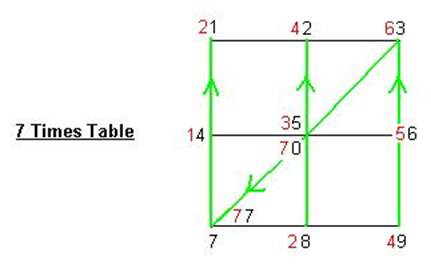The vector pattern is the same as that in the 3 times table, but in reverse direction. Starting from the 7 unit position on the square (1×7), the vectors point north, following the same route as to the 3 times table. The tens results (in red) written next to their associated unit positions, again, reveal a simple numerical progression.

The 9 times table follows the same vectors, rotated 90 degrees anti-clockwise: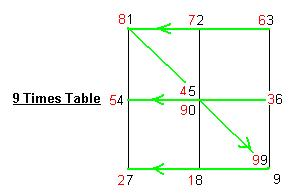Shown for the sake of mathematical completeness, the 1 times table follows the same course as the 9 times table, but in reverse: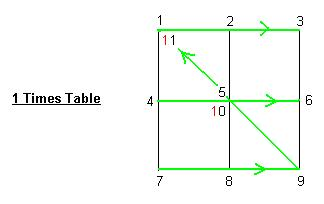[Note: I have not joined all the diagonal links with vectors, because it would be unnecessary and confusing. The aim is to simplify arithmetic functions not complicate them where it is not necessary.]

These multiplication tables (and here the use of the word “table” is perhaps more appropriate than ever it was before) reveal the vector patterns * for the 1, 3, 7 and 9 tables.

* [I use this word in its plural form. In reality there is only a single pattern with its vectors pointing in the same direction, for all 4 multiplication tables; it is simply rotated through 90 or 180 degrees.]

To complete the series of odd-numbered times tables, we should include the 5 times table. But this is harder to show as a vector pattern using the Decimal Square, as zero and 5 share the same number position at the centre. But if we separate the two positions and place them on a single line “framework”, like this: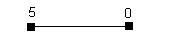We can now reveal the simplest pattern of all the multiplication tables: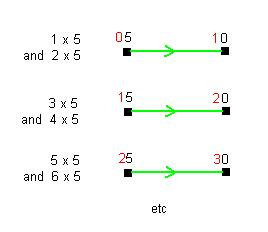The single line framework is, of course, the same line throughout the table, but I have shown it as separate lines to avoid confusion over the tens results.

To complete our investigations, we can use the same method to discover the vector patterns for the even-numbered times tables; 2, 4, 6 and 8.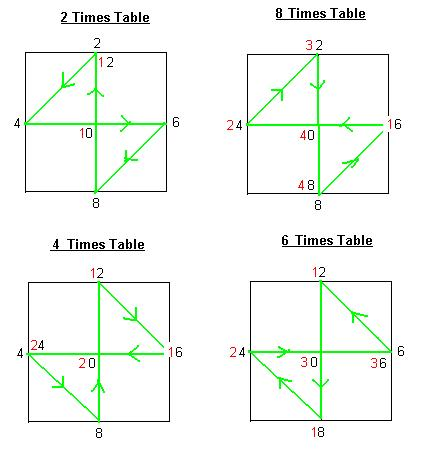So the 8 times table is the reverse of the 2 times table; and the 6 times table is the reverse of the 4 times table. All share the same pattern, but rotated through either 90 or 180 degrees.

Teachers using this system of Decimal Square Vector Times Tables, can show pupils that we can continue following the same vector patterns in any table far beyond the eleventh time (11 x n). Pupils can be set a challenge; what is 12 x , 13 x, 14 x the table being discussed.

Naturally, it is impossible for a short article such as this to provide a suggested framework for teaching the system in the curriculum. It can only be a brief presentation.

And I realize this is not a method for showing pupils the nature of multiplication – for example, as a number fact resulting from a series of additions of the same number. Instead, this system provides pupils with a method for obtaining the results of the times tables without the use of ʻcounting onʼ or rote memory; rather it relies on spatial, visual memory and the ability to visualize a planned sequence of events in 2 dimensional space using points, vectors and numerals. It also reveals a beautiful, unified symmetry that is the essence of mathematics.

——————————————————————————-